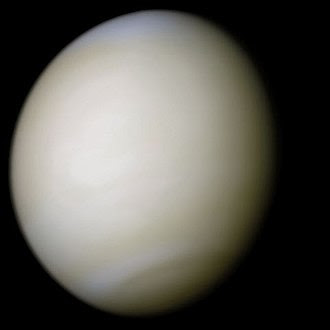### Biggest Planets in the Solar system

• Jupiter is the fifth planet from the Sun and it is the First Biggest Planet in the Solar system.
• Jupiter is the largest in the Solar System.
• It is a gas giant with a mass one-thousandth that of the Sun.
• But two-and-a-half times that of all the other planets in the Solar System combined.
• The mass of Jupiter is 1.898 × 10^27 kg (317.8 M⊕).
• The Orbital period of Jupiter is 12 years.
• The Radius of Jupiter is 69,911 km.
• The Surface area of Jupiter is 61.42 billion km².
• The Distance from Sun is 778.5 million km.
• The Moons for Jupiter are Europa, Ganymede, Io, Callisto, Valetudo, Amalthea, Carme, MORE...

## 2.♄SaturnSaturn
• It is the sixth planet from the Sun and it is the Second Biggest Planet in the Solar system.
• Saturn is a gas giant with an average radius of about 9 times that of Earth.
• Saturn only had one-eighth the average density of Earth.
• It is over 95 times more massive.
• The Orbital Period of Saturn is 29 years.
• The Distance from Sun is 1.434 billion km.
• The Mass of Saturn is 5.683 × 10^26 kg (95.16 M⊕).
• The Radius of Saturn is  58,232 km.
• The Surface area of Saturn is 42.7 billion km².
• The Moons for Saturn are Titan, Enceladus, Mimas, Iapetus, Dione, Hyperion, Phoebe, MORE...

## 3.⛢UranusUranus
• It is the seventh planet from the Sun.
• It has the third-largest planetary radius and it is the Third Biggest Planet in the Solar system.
• It has the fourth-largest planetary mass in the Solar System.
• It is similar in composition to Neptune.
• They both have bulk chemical compositions that differ from that of the larger gas giants Jupiter and Saturn.
• The Distance from Sun is 2.871 billion km.
• The Orbital period of Uranus is 84 years.
• Uranus is Discovered on 13 March 1781.
• The Mass of Uranus is 8.681 × 10^25 kg (14.54 M⊕).
• Uranus is Discovered by  William Herschel.
• The Moons for Uranus are Umbriel, Miranda, Titania, Oberon, Puck, Cressida, MORE...

## 4.♆NeptuneNeptune
• It is the eighth and farthest known planet from the Sun in the Solar System.
• In the Solar System, Neptune is the fourth-largest planet by diameter.
• The third-most-massive planet.
• Neptune is the densest giant planet.
• It is 17 times the mass of Earth and it is the Fourth Biggest Planet in the Solar system.
• Neptune is slightly more massive than its near-twin Uranus.
• The Orbital period of Neptune is 165 years.
• The Distance from Sun is 4.495 billion km.
• The Surface area of Neptune is 7.618 billion km².
• The Radius of Neptune is 24,622 km.
• The Moons for Neptune is Triton, Thalassa, Naiad, Nereid, Neso, Proteus, Hippocamp, Despina, Galatea, Halimede, Laomedeia, Psamathe, Larissa, Sao.
• The Discoverers of Neptune is Urbain Le Verrier, Johann Gottfried Galle, John Couch Adams.

## 5.♁EarthEarth
•  It is the 5th Largest Planet.
• The Orbital Period of Earth is  365.256363004 days.
• The Satellite Of the Earth is Moon and it is the Fifth Biggest Planet in the Solar system.
• The Mass of Earth is 5.97237×1024 kg (1.31668×1025 lb).
• The distance from the Sun is 149.60 million km.
• It is the only planet where there is life.
• The Age of Earth is 4.543 billion years.
• The Radius of Earth is 6,371 km.
• The Population of Earth is 759.43 crores (2018).

## 6.♀VenusVenus
• It is the second planet from the Sun.
• Venus is named after the Roman goddess of love and beauty.
• Venus is the second-brightest natural object in the night sky after the Moon.
• It can cast shadows and, rarely, is visible to the naked eye in broad daylight.
• The Orbital period of Venus is 225 days and it is the Sixth Biggest Planet in the Solar system.
• The Length of the day of Venus is  116d 18h 0m.
• The Distance from Sun is 108.2 million km.
• The Radius of Venus is 6,051.8 km.
• The Equatorial rotation velocity of Venus is  6.52 km/h (1.81 m/s).

## 7.♂︎MarsMars
• It is the fourth planet from the Sun.
• Mars is the second-smallest planet in the Solar System after Mercury.
• In English Mars means the name of the Roman god of war.
• It is often referred to as the 'Red Planet'.
• The Gravity of Mars is 3.711 m/s² and it is the Seventh Biggest Planet in the Solar system.
• The Orbital Period of Mars is 687 days.
• The Mass of Mars is 6.39 × 10^23 kg (0.107 M⊕).
• The surface pressure of Mars is 0.636 (0.4–0.87) kPa; 0.00628 atm.
• The Moons of Mars are Phobos, Deimos.

## 8.☿MercuryMercury
• It is the smallest and innermost planet in the Solar System.
• Mercury's orbit around the Sun takes 87.97 days.
• The shortest of all the planets in the Solar System.
• Mercury is named after the Roman deity Mercury.
• Mercury is the messenger of the gods and it is the Eighth Biggest Planet in the Solar system.
• The Orbital Period of Mercury is 88 days.
• The Distance from Sun is 57.91 million km
• The Length of a day of Mercury is 58d 15h 30m.
• The Mass of Mercury is 3.285 × 10^23 kg (0.055 M⊕).
• The Radius of Mercury is 2,439.7 km.